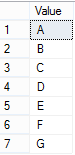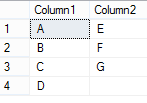# Monthly Archives: February 2015

## Divide rows of a column in two columns5

In this post “Divide rows in two columns”, we are going to learn a trick to divide a column’s rows in two columns. We have find the total number of rows and then distribute it in two columns, For example, a table with a column containing 6 rows, will split in two columns, each of 3 rows. In case of odd numbers of rows in the column, in second column, a blank string should appear for last entry. Lets have a look on the below table and required output to understand this;Required outputYou can see in above figures, we have 7 rows in our demo table and these rows have been distributed in two columns. Hence, we have a odd count for total rows, last row in column2 has a blank value. Below is the demo table with sample data.

In … More